### 10.1. The function of numerals

Numerals of the following types are distinguished:

#### 10.1.1. Numerals with the role of an attribute (`RSTR`)

When combined with a counted object, most numerals have the function of a restrictive attribute. The node for the counted noun is the governing node and the dependent node is the node representing the numeral. The node representing the numeral gets the `RSTR` functor.

Examples:

Mám pět.`RSTR` domů a tři.`RSTR` auta. (=I've got five houses and three cars) Fig. 8.178

naši tři.`RSTR` nejlepší hráči (=lit. our three best players)

Kolik.`RSTR` lidí přišlo? (=How many people came?)

náš druhý.`RSTR` nejlepší hráč (=our second best player)

Hodně.`RSTR` lidí se nechalo nalákat. (=Many people let themselves to be attracted)

The following classes of numerals are analyzed as dependent nodes with the `RSTR` functor when combined with a counted object:

1. definite cardinal numerals: "one" through "ninety-nine".

For example: jeden (=one), dva (=two), devadesát devět (=ninety-nine).

2. indefinite cardinal numerals with the exception of those that have the meaning of a "container"; see Section 10.1.2, "Numerals with the meaning of a "container"".

For example: několik (=several), tolik (=so many/much), kolik (=how many/much).

4. some other numerical expressions:

hodně (=a lot), více (=more), méně (=less), mnoho (=many/much), málo (=few/little), stejně (=as_much_as), plno (=plenty), dost (=enough).

When combined with a counted object, all the numerals under a) through d) are analyzed as adjectival (`sempos`=`adj.quant.def` or `adj.quant.indef`; see Section 6.2.4, "Definite quantificational semantic adjectives" and Section 6.2.5, "Indefinite quantificational semantic adjectives").

Annotation of numerals standing alone in a sentence (without a counted object). If a numeral from groups a) through d) stands alone in the sentence (without a counted object), it is analyzed according to which of the subgroups it belongs to:

• cardinal numerals, numerals from a) and b), are taken to be syntactic nouns. No new node (for a counted object) is inserted into the structure. The node representing the numeral can have different functors - also one of the functors for arguments.

!!! The `sempos` attribute of the node representing a definite cardinal numeral (group a) is assigned the value `n.quant.def`. As for the nodes representing indefinite cardinal numerals, no division into `adj.quant.indef` and `n.quant.indef` was made; all indefinite cardinal numerals (group b) have the attribute `sempos` specified as `adj.quant.indef` (i.e. even in cases without a counted object). See also Section 6.2.5, "Indefinite quantificational semantic adjectives".

Examples:

Zvolili tři.`PAT` z pěti místopředsedů. (=They elected three from five vice-chairs) Fig. 8.179

Mají několik druhů ovocných čajů. Tyhle tři.`ACT` jsou nejlepší. (=These three are the best)

Vezmi dva.`PAT` bílé. (=Take two of the white ones)

Kolik.`PAT` mi dáš? (=How much are you going to give me?)

Mám několik.`PAT` červených. (=I've got a couple of the red ones)

Počítá, kolik.`ACT` z vyřčených myšlenek odvane čas. (=He is counting how many of the uttered thoughts will be blown away by the passing time)

NB! In case there are several counted objects in coordination (or apposition) and in some of the conjoined expressions the counted object is omitted, a new node is inserted in the position of this non-expressed counted object, which is usually a copy of the node representing the expressed counted object.

These cases are not understood as cases of numerals standing alone (i.e. numerals with the nominal function) but as cases of ellipsis.

Examples:

Má 10 tuzemských jogurtů a 5.`RSTR` { jogurt.`PAT`} cizích. (=He's got ten yoghurts produced here and five from abroad) Fig. 8.181

Vystudoval 8 tříd, čtyři.`RSTR` { třída.`PAT`} rumunské, čtyři.`RSTR` { třída.`PAT`} české. (=He finished eight classes, four Romanian, four Czech) Fig. 8.182

• adjectival numerals and numerals from group c) and d) are analyzed as syntactic (and semantic) adjectives (`sempos`=`adj.quant.def` or `adj.quant.indef`; see Section 6.2.4, "Definite quantificational semantic adjectives" and Section 6.2.5, "Indefinite quantificational semantic adjectives") also in cases they stand alone (without a counted object) in a sentence.

A new node for the omitted governing noun is added to the tectogrammatical tree in such cases, following the rules in Section 12.1.2, "Ellipsis of the governing noun".

Examples:

Třetí.`RSTR` {`#EmpNoun`.`PAT`} už jsme nestihli. (=We didn't make it for the third one) Fig. 8.180

Třetí.`RSTR` {`#EmpNoun`.`ACT`} už tu není. (=The third one is not here anymore)

hodně.`RSTR` {`#EmpNoun`.`PAT`} (=She's got a lot)

No node for the governing noun (counted object) is inserted, if the numeral is:

• in the position of the Patient or Effect and it agrees with another valency modification (the Patient or Effect position corresponds to a predicative complement here):

Examples:

Zůstal třetí.`PAT` (=He remained third)

Pokládali ho za druhého.`EFF` v pořadí. (=They considered him the second)

• in the position of the nominal (non-verbal) part of a verbonominal predicate (see Section 2.1.3, "Copula "být" (verbonominal predicate)"):

Example:

To je druhé.`PAT` (=This is the second)

• in the position of a predicative complement (`COMPL`; see Section 10, "Predicative complement (dual dependency)").

Example:

Pavel skončil jako druhý.`COMPL` (=Pavel took second place, lit. finished as second)

Another example:

Máme dvě.`RSTR` možnosti. Jedna.`ACT` spočívá v pasivním vyčkávání. Druhá.`RSTR` { možnost.`ACT`} předpokládá aktivní přístup. (=We've got two possibilities. One is.. The second presupposes...)

Figure 8.178. Numerals with the role of an attribute (`RSTR`)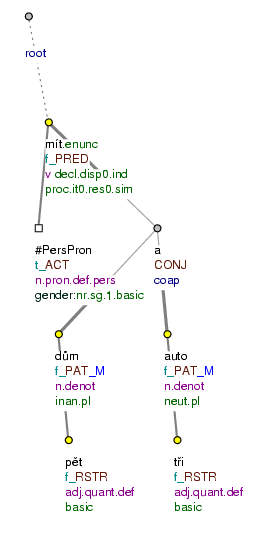Mám pět domů a tři auta. (=lit. (I) have five houses and three cars)

Figure 8.179. Cardinal numerals standing alone (without a counted object)Zvolili tři z pěti místopředsedů. (=lit. (They) elected three out_of five vice-chairs)

Figure 8.180. Adjectival numerals standing alone (without a counted object)Třetí už jsme nestihli. (=lit. Third (we) already AUX missed)

Figure 8.181. Counted objects in coordination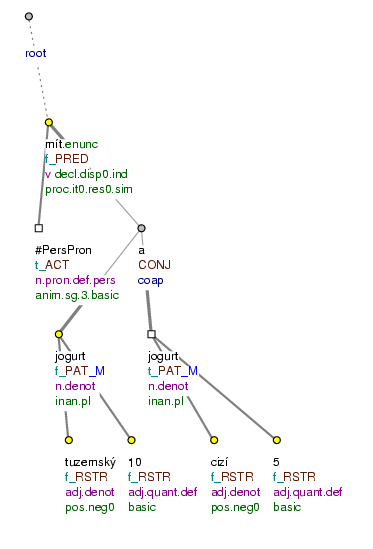Má 10 tuzemských jogurtů a 5 cizích. (=lit. (He) has 10 domestic yoghurts and 5 foreign)

Figure 8.182. Counted objects in coordination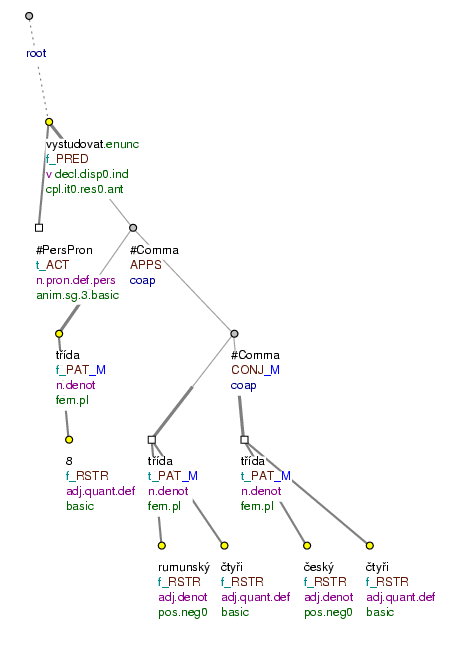Vystudoval 8 tříd, čtyři rumunské, čtyři české. (=lit. (He) finished 8 classes, four Romanian, four Czech)

#### 10.1.2. Numerals with the meaning of a "container"

Distinct from the numerals that have the function of an attribute (`RSTR`) when combined with a counted object, there are also numerals that have the function (meaning) of a container in the same environment (cf. the functor `MAT` - Section 10.4, "MAT"). Numerals with the meaning of a container are syntactic (hence semantic) nouns (`sempos`=`n.quant.def`; see Section 6.1.5, "Definite quantificational semantic nouns").

When such a numeral is combined with a counted object, the governing node is the node representing the numeral and the node for the counted object depends on it. The node representing the counted object gets the `MAT` functor.

The following numerals are considered numerals with the meaning of a container:

• milion (=million), (and other numerals ending in -ion), miliarda (=billion), polovina (=half), polovice (=half), půl(e) (=half), třetina (=(one) third), čtvrt (=(one) fourth/quarter), čtvrtina (=(one) fourth), tisícina (=(one) thousandth), tucet (=dozen), veletucet (=twelve dozen), kopa (=five dozen/heap), řada (=row), spousta (=a lot), hromada (=heap/pile), zástup (=crowd/multitude), dav (=crowd), dvojice (=couple), trojice (=triple), sto (=hundred), tisíc (=thousand), trocha/u (=a bit).

In cases when a numeral with the meaning of a container is a counted object at the same time, the annotation differs depending on whether the first (i.e. the "counting") numeral does or does not have the meaning of a container, too. Cf.:

• Žije tu jeden.`RSTR` milion lidí.`MAT` (=One million people live here)

The numeral milión is a counted object simultaneously. The (counting) numeral jeden does not have the meaning of a container. The annotation follows the rules in Section 10.1.1, "Numerals with the role of an attribute (`RSTR`)". Cf. Fig. 8.183.

• Ani čtvrt milionu.`MAT` dolarů.`MAT` by na to nestačilo. (=Not even a quarter of a million dollars would do)

The numeral milión is a counted object at the same time. The (counting) numeral čtvrt has the meaning of a container. The annotation follows the rules described in the present section. Cf. Fig. 8.184.

More examples:

Po půl roce.`MAT` se všechno změnilo. (=After half a year everything changed)

První.`RSTR` polovina června.`MAT` byla studená. (=The first half of June was cold)

Řada lidí.`MAT` se ale domnívá pravý opak. (=A number of people think the exact opposite)

s celým stem lidí.`MAT` (=with hundred people)

byly tam dva.`RSTR` tisíce lidí.`MAT` (=There were two thousand people there)

dvojice kanoistů.`MAT` (=lit. couple/pair (of) canoeists) (but: dvojice Mach.`ID` a Šebestová.`ID` (=lit. couple Mach and Šebestová); see also Section 8.2.1, "Specific rules for certain types of proper nouns")

!!! Cases like s čtvrt milionem lidí (=with a quarter of million people), se sto lidmi (=with hundred people.INSTR), s trochou lidmi (=with a couple of people.INSTR) were (when they emerged) analyzed on a par with cases when the counted object is in the genitive (the node representing the counted object gets the `MAT` functor).

Figure 8.183. Numerals with the meaning of a "container"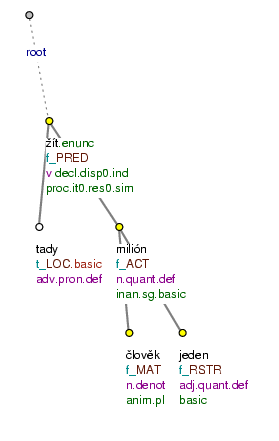Žije tu jeden milion lidí. (=lit. Lives here one million people)

Figure 8.184. Numerals with the meaning of a "container"Ani čtvrt milionu dolarů by na to nestačilo. (=lit. Not_even quarter million dollars would for it not_be_enough)

#### 10.1.3. Numerals with the function of a "label"

Definite cardinal numerals (especially those written in digits) often have the function of a "label". This concerns numerals used for labelling (numbering) objects (e.g. phone/fax numbers, house numbers, post codes, serial numbers, numbers in names of products).

Numerals with the function of a label are syntactic (hence semantic) nouns.

!!! The numerals have the `n.quant.def` value in the `sempos` attribute (see Section 6.1.5, "Definite quantificational semantic nouns") if they follow these expressions: rok (=year), číslo (=number), telefon (=telephone), fax, tel., PSČ (=post code), paragraf (=article), odstavec (=paragraph), odst. (=para), č. (=No), sbírka (=code of law), zákon (=law), vyhláška (=regulation), sezona (=season), \$. If they follow other expressions, the value of the attribute is `adj.quant.def`.

The numerals with the label function are assigned a functor according to the rules for identifying expressions described mainly in Section 8, "Identifying expressions"; in addresses, the numerals usally get the `RSTR` functor, see Section 12.2, "Addresses".

Examples:

Nový Golf 500.`RSTR` už je na trhu. (=The new Golf 500 is already on the market) Fig. 8.185

Bydlí v ulici Strmá 34.`RSTR` (=He lives in Strmá 34) Fig. 8.186

Výstavu organizuje Sdružení pro obnovu a rozvoj lidových řemesel a tradic, Vojtěšská l3.`RSTR` , Praha 1.`RSTR` , tel.: ( 02 ) 291 591.`RSTR` (=The exhibition is organized by the Association ..., Vojtěšská 13...)

Čtete na str. 12.`RSTR` (=You're reading on page 12)

A vzhledem k tomu, že Škodovka zvyšuje ceny rychleji, než jsme očekávali, není daleko doba, kdy se dostaneme k číslu 10 000.`RSTR` (=...we'll soon get to number 10 000)

Figure 8.185. Numerals with the function of a "label"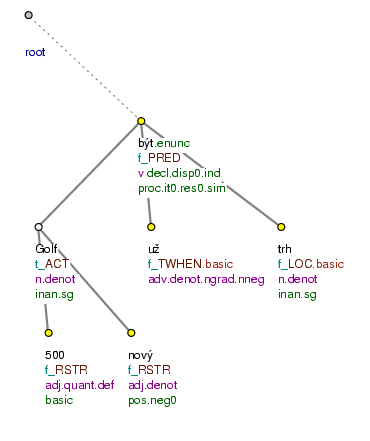Nový Golf 500 už je na trhu. (=lit. New Golf 500 already is on market)

Figure 8.186. Numerals with the function of a "label"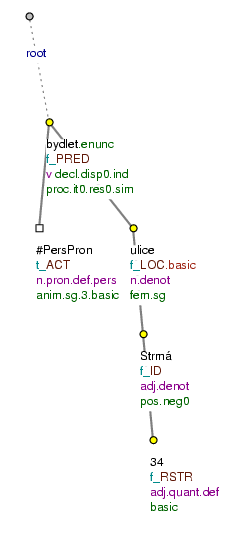Bydlí v ulici Strmá 34. (=lit. (She) lives in street Strmá 34)

#### 10.1.4. Numerals with adverbial meanings

Nodes representing numerals like pětkrát (=five times), několikrát (=several times), jednou (=once), podruhé (=for the second time) etc., which express various adverbial meanings, get a functor corresponding to their position in the sentence (adjunct functors).

Examples:

Vyhráli jsme jen dvakrát.`THO` (=We only won twice) Fig. 8.187

Podruhé.`TWHEN` už to neudělám. (=I'm not going to do it for the second time)

Třikrát.`THO` měř, jednou.`THO` řež. (=lit. Three times measure, once cut; meaning: think twice)

Figure 8.187. Numerals with adverbial meanings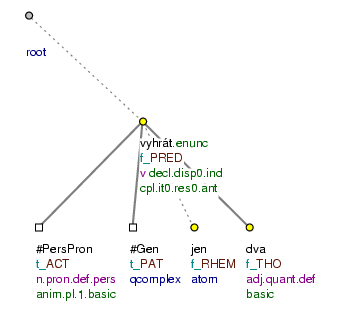Vyhráli jsme jen dvakrát. (=lit. (We) won AUX only twice)